×

Search anything:

# Sethi Ullman algorithm

#### Compiler Design AlgorithmsFREE BOOK -> Problems for the day before your Coding Interview (on Amazon)

The Sethi-Ullman algorithm is an optimal algorithm used for finding an optimal ordering of a computation tree. We will see two generalizations that increase its applicability without increasing the cost.

1. Introduction.
2. Generalization 1.
3. Generalization 2.
4. An evaluation algorithm.
5. Spilling registers.
6. Implementation.
7. Summary.
8. References.

## Introduction.

Computations can be modeled as computation trees whereby the leaves are constant values while the internal nodes acquire their values inductively as a specified arithmetic function of the values of their children.

An example
Given an expression: (3 + (4 + 3) x (5 + 6), we have the following tree;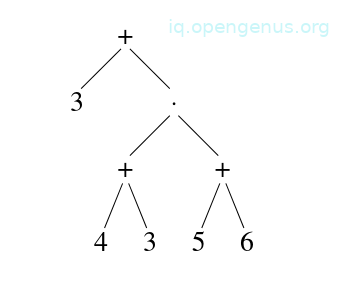On a machine, this computation is evaluated by storing values of previously computed nodes in registers and when a previously computed child node is needed to compute its parent node's value, the register associated with the child is re-used.

The Sethi-Ullman algorithm comes into play when we need to compute the nodes of a tree that use the fewest possible registers which are subject to the following assumptions:

1. No arithmetic identities are used.
2. All registers are equal
3. The tree is binary.
4. The value of a node fits in a single register.

This algorithm relies on an observation that once a computation begins, it is better to complete it before moving to a disjoint subtree and therefore it decides which child to evaluate first for each node.

If an optimally ordered computation of the left subtree needs n registers and the right subtree needs m registers, we have three cases that we consider to determine the number of registers needed:

1. n > m: we first compute the left child using n registers, finally we free all registers except the one holding the left child node value after which we compute the right child using m registers. Finally, we compute the parent. In this whole process, not more than n registers were used.
2. n < m: This is similar to the above case - we compute the right child first.
3. n = m: Either children can be computed the first followed by the other child. Here we use n+1 registers because the second child's computation takes place while there is a register holding the value of the first child.

In the above cases, nodes are labeled with a Sethi-Ullman number which represents the number of registers needed for its computation which is equal to the number of its children(if they are equal) or the number of either children + 1(not equal).

This is said to take O(n) time.

This, however, is restrictive in real compilers so we discuss two generalizations that are easy to implement.

## Generalization 1.

This generalization involves removing the restriction on the degree of nodes.

An example:
We have the instruction:

It computes a value from the values of several registers and constants.

It computes the value of the tree below in register 5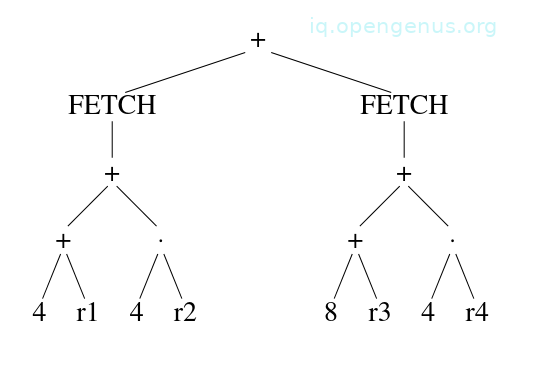We can model it as a 4-ary node as follows: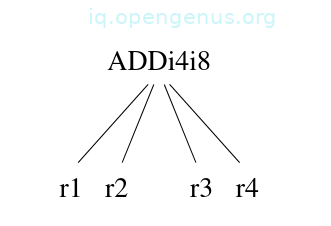## Generalization 2.

This involves removing the restriction on the size of the computed result. On many computers, operands e.g double-precision, floating-points, etc, require two registers to store them.
Each tree node should be annotated with the number of registers that store the value computed by the node.

An example:
Consider the VAX addressing modes, the operands of add instructions may be complex and use more registers as shown below: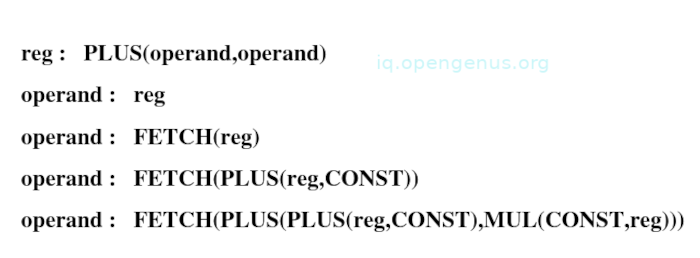Where the operand types correspond to register mode, register-deferred mode, displacement-deferred mode, and displacement-deferred-indexed mode respectively.
The operand value requires two registers to store it.
The last of the operand pattern specifies the displacement-deferred-indexed mode for example;
4(r1)[r5]

Now, to incorporate the above into an instruction, we accumulate other operands while still holding the values of registers 1 and 5.

The addressing mode works with any pair of registers therefore they don't have to be adjacent to each other. This is important since the allocation for a multi-register value is easier when multiple-registers may be any subset of the register bank.

This way, we extend the model and include more trees with multiple children and nodes that need more than one register to hold their result.

This generalization also includes simple cases e.g we have the pattern:
operand: CONST that corresponds to an intermediate-mode operand, for this no registers are needed to hold its value since it is compiled directly into an instruction and has no subtrees.

## An evaluation algorithm.

We need an algorithm that minimizes the number of registers needed subject to the constraint that one subtree's evaluation is completed before the disjoint subtree's evaluation starts.

For each node t we have as input the number ${\mathrm{b}}_{\mathrm{t}}$ of registers needed to hold t's result.
We compute the number ${\mathrm{a}}_{\mathrm{t}}$ of registers that are needed for this evaluation.
Assuming we have node t with k children and have computed for each child i the number ${\mathrm{a}}_{\mathrm{i}}$.
We find a permutation ${\mathrm{\pi }}_{\mathrm{min}}$ such that the evaluation of the children in the order ${\mathrm{\pi }}_{\mathrm{min}}$(1), ${\mathrm{\pi }}_{\mathrm{min}}$(2), ..., ${\mathrm{\pi }}_{\mathrm{min}}$(k) takes the minimum number of registers.

The number of registers for a permutation π is given by;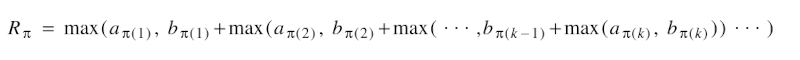The reasoning behind the above equation is as follows, the subtree π(1) is first evaluated and this needs ${\mathrm{a}}_{\mathrm{\pi \left(1\right)}}$ registers, then, the remaining subtrees are evaluated while storing the result of subtree π(1) in ${\mathrm{b}}_{\mathrm{\pi \left(1\right)}}$ registers and this requires a number of registers equal to the sum of ${\mathrm{b}}_{\mathrm{\pi \left(1\right)}}$ and the number of registers that are needed to compute the rest of the children.

Since the number of registers needed to compute the parent is larger than ${\mathrm{a}}_{\mathrm{\pi \left(1\right)}}$ and the sum, we compute ${\mathrm{a}}_{\mathrm{t}}$ as the minimum of ${\mathrm{R}}_{\mathrm{\pi }}$ over all permutations π.

Now, to find an optimal permutation is no more difficult than sorting k numbers, that is, we sort the ordered pairs (${\mathrm{a}}_{\mathrm{i}}$, ${\mathrm{b}}_{\mathrm{i}}$) by their differences to obtain an optimal ordering.

Therefore we have the following theorem:
Theorem: Each permutation ${\mathrm{\pi }}_{\mathrm{min}}$ that satisfies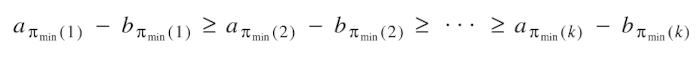minimizes R.

The proof of the theorem can be found in the original paper, the link is provided in the references section.

## Spilling registers.

When the number of registers needed exceeds the number of available registers, some values are 'spilled' into memory.
The original algorithm spills values during the evaluation of any node whose label is greater than the available registers r.

Let n be the number of registers needed in computing the left subtree and m be the number of registers required to compute the right subtree.

Now we have two cases:
m < n ≤ 1: if so, we compute the left child first.
n < m ≤ 1: in this case we compute the right child first,
Otherwise: the computation is performed in registers. Here, we evaluate either subtree first and save its value in memory followed by the other subtree then fetch the saved value and proceed with the computation.

The generalized version of the spilling algorithm involves sorting the nodes of the subtree into a list of nodes ${\mathrm{n}}_{\mathrm{i}}$ after which they are evaluated. At this point enough registers will be saved in memory and thus evaluation of the rest of the list is possible.

When all ${\mathrm{n}}_{\mathrm{i}}$ nodes have been evaluated, the saved values are fetched before operating on the root node. This is dependent on the value of ${\mathrm{b}}_{\mathrm{i}}$ not being greater than r. Assuming this is the case, then there is a machine that needs more registers than there are.

## Implementation.

The generalized algorithm is useful for an automatic code generator for the following reasons:

1. Such a system will process nodes of varying degrees.
2. Automatically generated code easily takes advantage of the index modes that lead to nodes with multi-register values

The generalized Sethi-Ullman ordering procedure.

``````struct node{
int hold;
int maxregs;
int sideEffect;
. . . other fields
};

int reorder(nodes) struct node *nodes[];{
int i, j;
struct node *temp;

for(j = 0; nodes[j] != NULL; j++)
for(i = j; i > 0; i--)
if(nodes[i]->maxregs - nodes[i]->hold
> nodes[i-1]->maxregs - nodes[i-1]->hold
&& !(nodes[i]->sideEffect || nodes[i-1]->sideEffect)){
temp = nodes[i];
nodes[i] = nodes[i-1];
nodes[i-1] = temp;
}
maxregs = 0;
for(i = j - 1; i >= 0; i--){
maxregs += nodes[i]->hold;
if(maxregs < nodes[i]->maxregs)
maxregs = nodes[i]->maxregs;
}
return maxregs;
}
``````

The procedure above computes the optimal ordering and the number of registers that are required to evaluate the children of a node.

The hold field of a node specifies the number of registers that are needed to hold its computed result.
The max-regs field specifies the number of registers needed at some point during computation. The procedure sorts the list using insertion sort according to this value.
The sideEffect field is a boolean flag that specifies whether a subtree rooted at a node has side effects e.g assignment statements, if so, it is unsafe to move the evaluation of such a node from its original position.
Parameter nodes is a NULL-terminated vector of node pointers.

After nodes are sorted into an optimal evaluation ordering, the parent node's maxregs value is computed and returned.

## Summary.

By having a generalized Sethi-Ullman algorithm, it is possible to have a simple procedure that performs tree-reordering rather than have multiple special cases and heuristics spread out in the code generator.

By incorporating this algorithm into a compiler we add to its size and execution time just by a small margin.

1. Original paper titled "Generalizations of the Sethi-Ullman algorithm for register allocation" (PDF) by Andrew W. Appel and Kenneth J. Supowit of Princeton University.

#### Erick Lumunge

Erick is a passionate programmer with a computer science background who loves to learn about and use code to impact lives positively.

Sethi Ullman algorithm